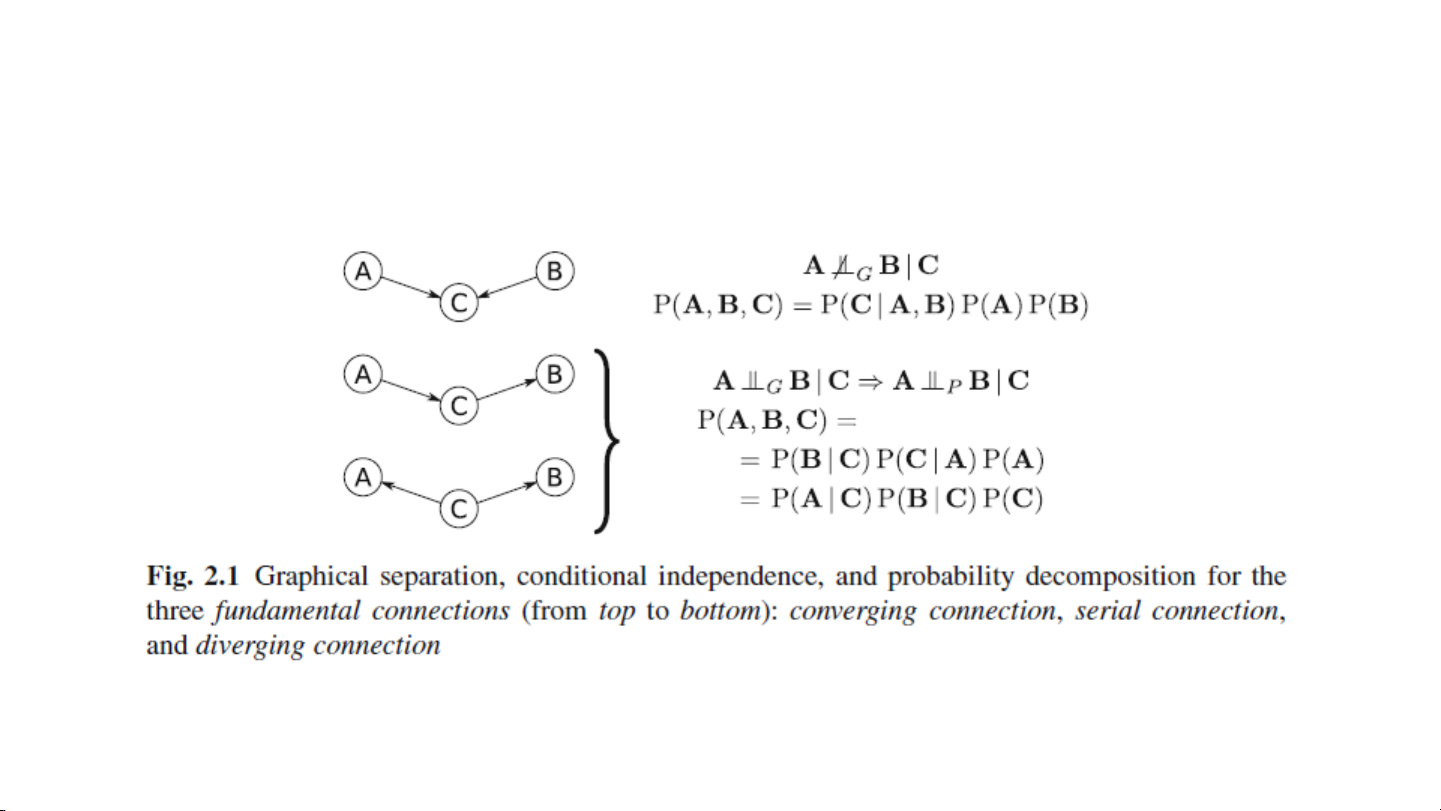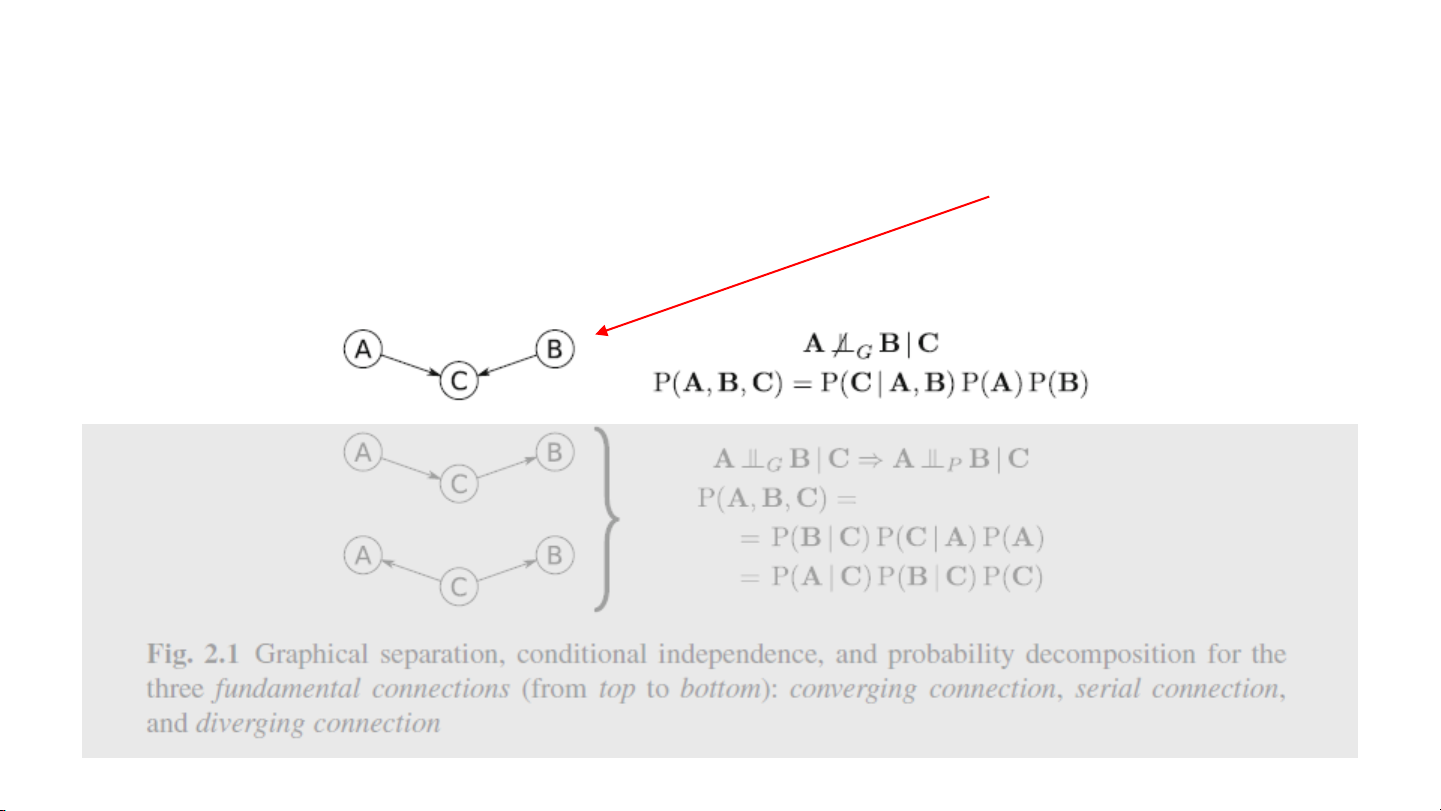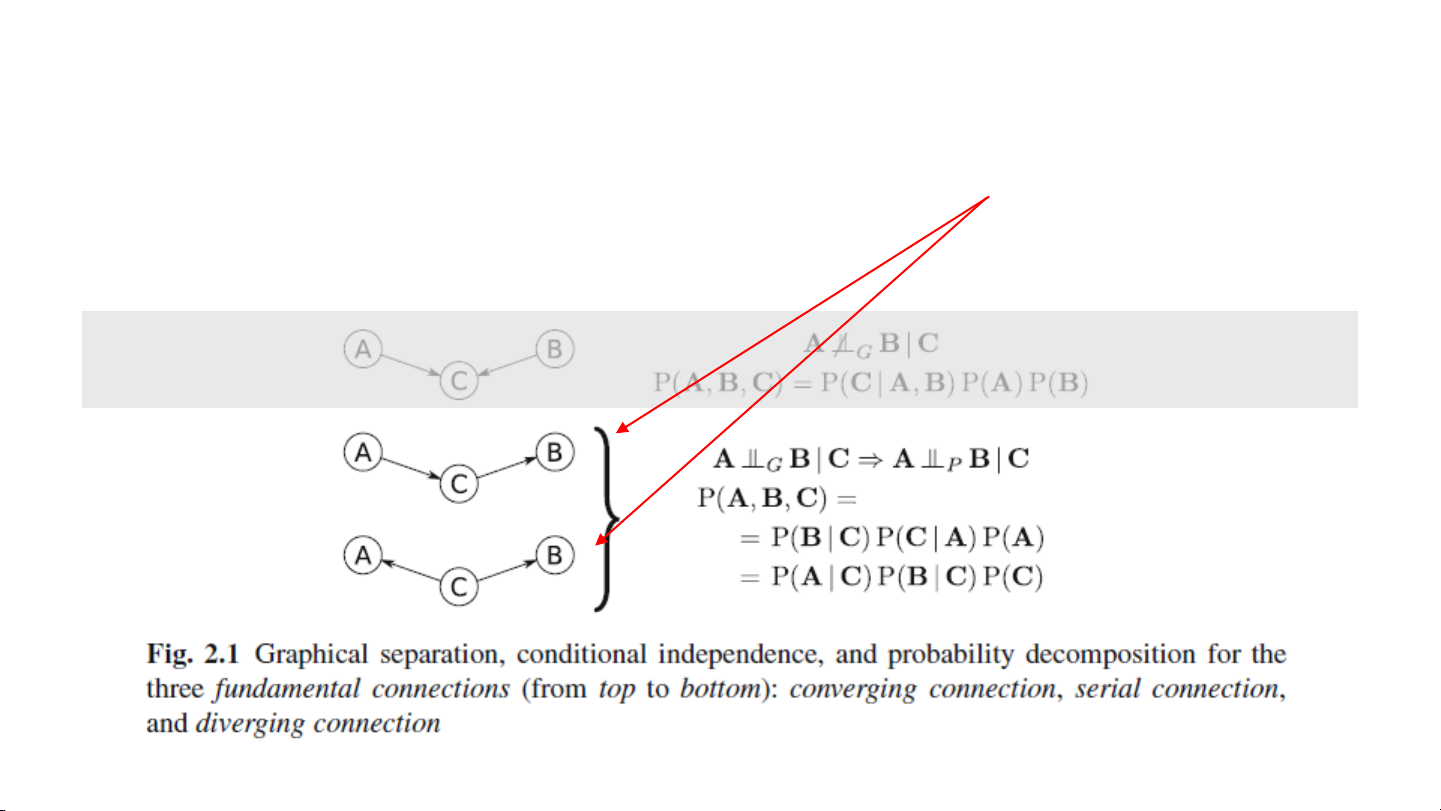Chapter 2 - Static Bayesian Networks:
Bayesian Networks in the Absence of
Temporal InformationStatic Bayesian networks, or Bayesian networks are:
graphical models that allow a concise representation of the probabilistic dependencies
between a given set of random variables
X = {X1,X2, . . . ,Xp}
as a DAG G = (V,A) where each node corresponds to a random variable Xi.
2.1.1 Graph structure
Independence map - because there is no probabilistic independence, an Independence map (I-
map) is the probabilistic dependence structure P of X if there is a on-to-one correspondence
between the random variables in X and the nodes V of G2.1.1 Fundamental connections2.1.2 D- separation
V- structure: A and B are not independent given C2.1.3 Equivalent structures.
Serial and diverging: A and B are independent
given C